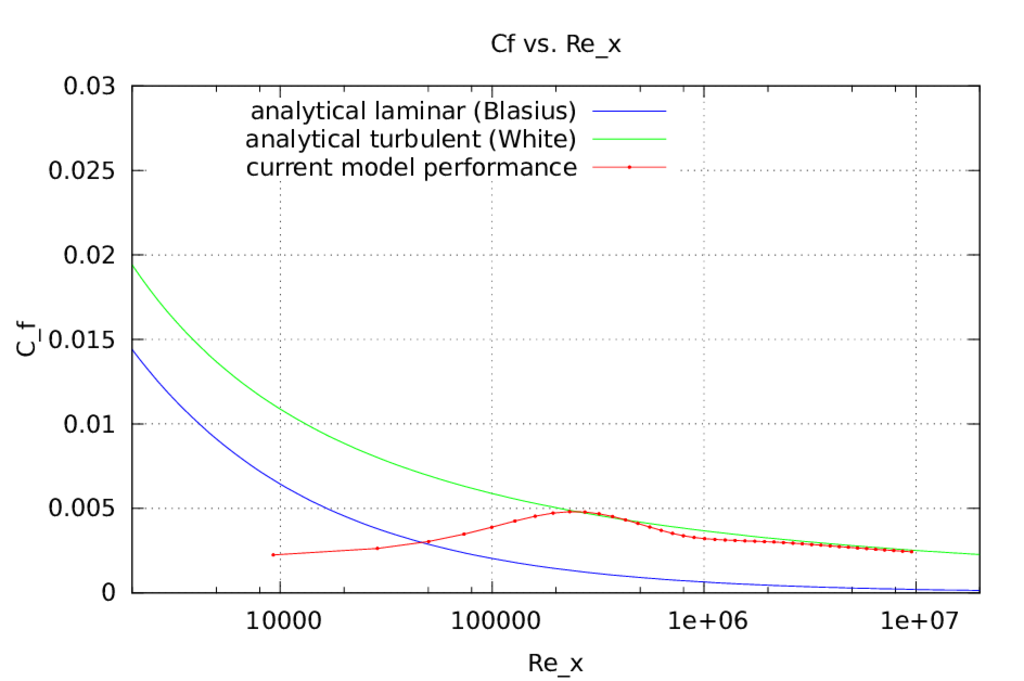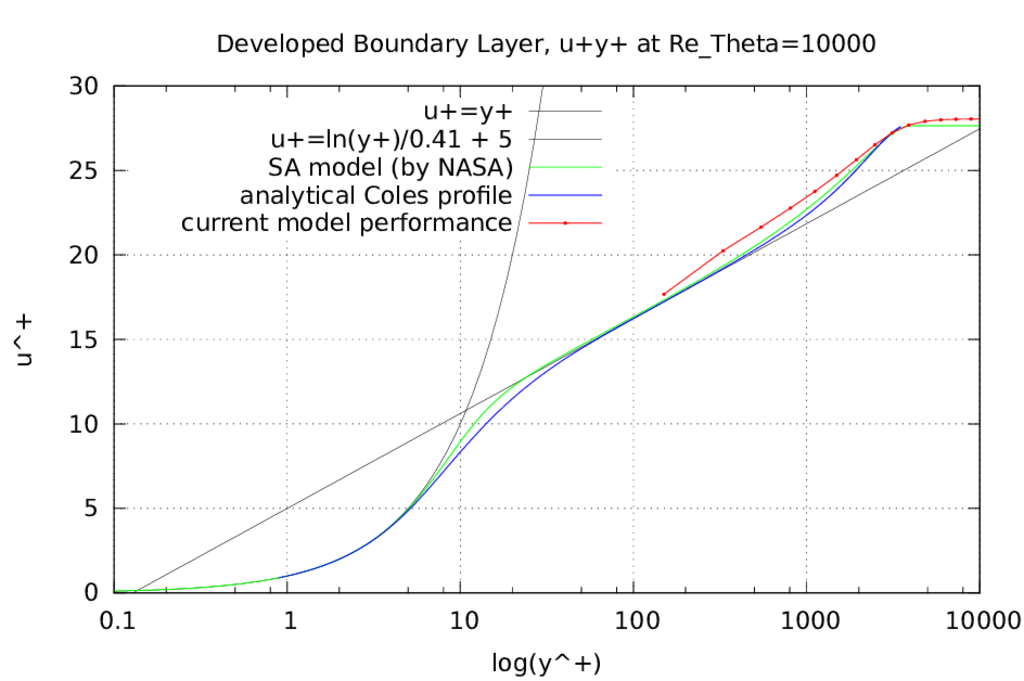Previous: RANS, model, lowRe (reolved Up: Flat Plate Test Case Next: NACA4412 Test Case

This is an automatically generated documentation by LaTeX2HTML utility. In case of any issue, please, contact us at info@cfdsupport.com.

# RANS,model, wall functions

The run script Allrun.sh settings is following:

turbulence=RANS
solver=simpleFoam
mode=incompressible
wall=wallFunction
endTime=0.9
deltaT=0.0001
NProc=6
Nx=50
Ny=30
U=50;
turbulenceModel=kOmegaSST
yGrading=200
Figure: $k$ $-$ $\omega$ $SST$ turbulence model. Wall functions. Mesh size 50×30. Friction coefficient $C_f$ versus Reynolds based on x coordinate $Re_x$ at the flat plate. Equations ([*]) show experimental correlation of various measurements. For laminar fluid flows at the flat plate the Blasius formula is used. For turbulent fluid flows at the flat plate the White formula is used.Figure:turbulence model. Wall functions. Mesh size 50×30. Friction coefficientversus Reynolds based on momentum thicknessat the flat plate. The comparison data are from Karman-Schoenherr formula and NASA simulation (Spalart-Allmaras model)Figure:turbulence model. Wall functions. Mesh size 50×30. Developed turbulent boundary layer in u+ and y+ coordinates at point whereThe comparison data are from Coles velocity law and NASA simulation (Spalart-Allmaras model)